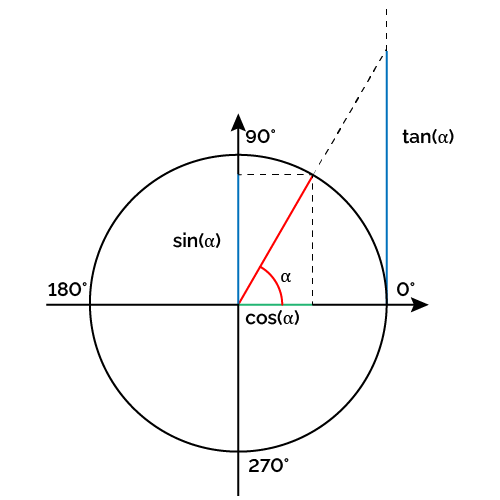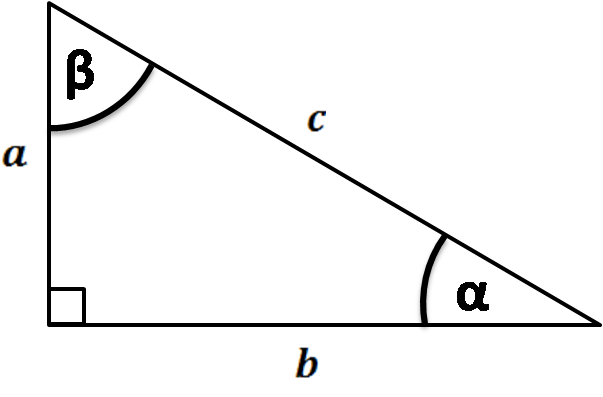# Right Triangle Trigonometry Calculator

Created by Davide Borchia
Reviewed by Luis Hoyos
Last updated: Jun 05, 2023

The right triangle trigonometry calculator can help you with problems where angles and triangles meet: keep reading to find out:

• The basics of trigonometry;
• How to calculate a right triangle with trigonometry;
• A worked example of how to use trigonometry to calculate a right triangle with steps;

And much more!

## Basics of trigonometry

Trigonometry is a branch of mathematics that relates angles to the length of specific segments. We identify multiple trigonometric functions: sine, cosine, and tangent, for example. They all take an angle as their argument, returning the measure of a length associated with the angle itself. Using a trigonometric circle, we can identify some of the trigonometric functions and their relationship with angles.As you can see from the picture, sine and cosine equal the projection of the radius on the axis, while the tangent lies outside the circle. If you look closely, you can identify a right triangle using the elements we introduced above: let's discover the relationship between trigonometric functions and this shape.

## Right triangles trigonometry calculations

Consider an acute angle in the trigonometric circle above: notice how you can build a right triangle where:

• The radius is the hypotenuse; and
• The sine and cosine are the catheti of the triangle.

$\alpha$ is one of the acute angles, while the right angle lies at the intersection of the catheti (sine and cosine)

Let this sink in for a moment: the length of the cathetus opposite from the angle $\alpha$ is its sine, $\sin(\alpha)$! You just found an easy and quick way to calculate the angles and sides of a right triangle using trigonometry.

The complete relationships between angles and sides of a right triangle need to contain a scaling factor, usually the radius (the hypotenuse). Identify the opposite and adjacent. We can then write:

$\begin{split} \sin(\alpha)&= \frac{\mathrm{opposite}}{\mathrm{hypotenuse}}\\[1em] \cos(\alpha)&= \frac{\mathrm{adjacent}}{\mathrm{hypotenuse}}\\[1em] \tan(\alpha)&= \frac{\mathrm{opposite}}{\mathrm{adjacent}}\\[1em] \end{split}$

By switching the roles of the legs, you can find the values of the trigonometric functions for the other angle.

Taking the inverse of the trigonometric functions, you can find the values of the acute angles in any right triangle.

Using the three equations above and a combination of sides, angles, or other quantities, you can solve any right triangle. The cases we implemented in our calculator are:

• Solving the triangle knowing two sides;
• Solving the triangle knowing one angle and one side; and
• Solving the triangle knowing the area and one side.

## Example of right triangle trigonometry calculations with steps

Take a right triangle with hypotenuse $c = 5$ and an angle $\alpha=38\degree$. Surprisingly enough, this is enough data to fully solve the right triangle! Follow these steps:

1. Calculate the third angle: $\beta = 180\degree - \alpha$.
2. Calculate the sine of $\alpha$ and use its value to find the length of the opposite cathetus:
• $\sin(\alpha) = 0.61567$.
• $\mathrm{opposite} = \sin(\alpha)\cdot\mathrm{hypotenuse} = 0.61567 \cdot 5 = 3.078$.
3. Find the length of the last side by either using Pythagoras' theorem or the cosine relations $\cos(\alpha) = \mathrm{adjacent}/\mathrm{hypotenuse}$. Given $\cos(\alpha) =0.788$:
• $\mathrm{adjacent} = 0.788\cdot 5 = 3.94$.

That's it!

## More trigonometry and right triangles calculators (and not only)

If you liked our right triangle trigonometry calculator, why not try our other related tools? Here they are:

## FAQ

### How do I apply trigonometry to a right triangle?

To apply trigonometry to a right triangle, remember that sine and cosine correspond to the legs of a right triangle. To solve a right triangle using trigonometry:

1. Identify an acute angle in the triangle α. For this angle:
• sin(α) = opposite/hypotenuse; and
• cos(α) = adjacent/hypotenuse.
2. By taking the inverse trigonometric functions, we can find the value of the angle α.
3. You can repeat the procedure for the other angle.

### What is the hypotenuse of a triangle with α = 30° and opposite leg a = 3?

The length of the hypotenuse is 6. To find this result:

1. Calculate the sine of α: sin(α) = sin(30°) = 1/2.
2. Apply the following formula:
sin(α) = opposite/hypotenuse
hypotenuse = opposite/sin(α) = 3 · 2 = 6.

That's it!

### Can I apply right-triangle trigonometric rules in a non-right triangle?

Not directly: to apply the relationships between trigonometric functions and sides of a triangle, divide the shape alongside one of the heights lying inside it. This way, you can split the triangle into two right triangles and, with the right combination of data, solve it!

Davide Borchia
Given
two sides
a
in
b
in
c
in
Angle α
deg
Angle β
degPeople also viewed…

### Free fall

Our free fall calculator can find the velocity of a falling object and the height it drops from.

### Greatest common factor

This greatest common factor calculator can find the GCF of a set of numbers.

### Humans vs vampires

Vampire apocalypse calculator shows what would happen if vampires were among us using the predator - prey model.

### Percentage

Simple percentage calculator is a generic percentage-based problem solver.